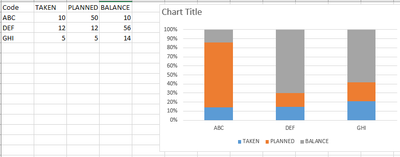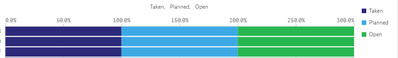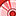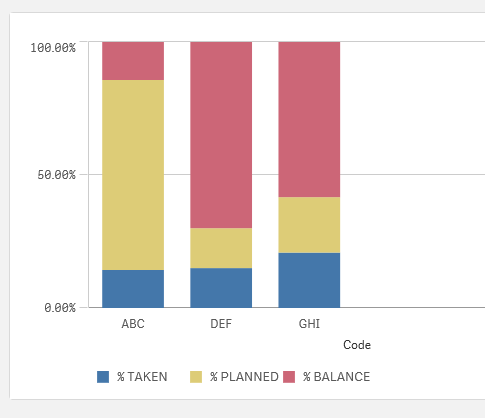# New to Qlik Sense

If you’re new to Qlik Sense, start with this Discussion Board and get up-to-speed quickly.

Announcements
LINKEDIN LIVE: Democratizing data to enhance customer-centricity. JULY 29TH REGISTER TODAY
cancel
Showing results for
Did you mean:Contributor III

## 100% Stacked Bar Chart using multiple measures

i am trying to plot a 100% stacked bar chart, showing the variance across three categories for each group and it was relatively automatic and easy in excel to plot ..but when i tried to same in qlik sense .. this is what i am getting, i tried using total function but still no luck.

sum([Taken])/sum(TOTAL<Group> [taken])

sum([Planned])/sum(TOTAL<Group> [Planned])

sum([Open])/sum(TOTAL<Group> [Open])Labels (4)

• ### Qlik Sense

1 Solution

Accepted SolutionsSpecialist II

Using the following data:

Data:
Code,TAKEN,PLANNED,BALANCE
ABC,10,50,10
DEF,12,12,56
GHI,5,5,14
];

Formula for % TAKEN

=Sum(TAKEN)/(SUM(TAKEN)+SUM(PLANNED)+SUM(BALANCE))

Formula for % PLANNED

=Sum(PLANNED)/(SUM(TAKEN)+SUM(PLANNED)+SUM(BALANCE))

Formula for % BALANCE

=Sum(BALANCE)/(SUM(TAKEN)+SUM(PLANNED)+SUM(BALANCE))

Set the presentation to be a stacked  bar chart2 RepliesSpecialist II

Using the following data:

Data:
Code,TAKEN,PLANNED,BALANCE
ABC,10,50,10
DEF,12,12,56
GHI,5,5,14
];

Formula for % TAKEN

=Sum(TAKEN)/(SUM(TAKEN)+SUM(PLANNED)+SUM(BALANCE))

Formula for % PLANNED

=Sum(PLANNED)/(SUM(TAKEN)+SUM(PLANNED)+SUM(BALANCE))

Formula for % BALANCE

=Sum(BALANCE)/(SUM(TAKEN)+SUM(PLANNED)+SUM(BALANCE))

Set the presentation to be a stacked  bar chartPartner

Here's another solution. It might not be suitable, but it's good to know that it's available.

Use crosstable to convert the three columns into another dimension and then you have two dimensions and only one measure on your stacked bar chart.Then you only need one formula:

=Sum(Value) / sum( total<Code> Value )Barnaby Dunn
BI ConsultantTags
Community Browser